Function Repository Resource:

# MannKendallZ

Compute the z-statistic for the Mann-Kendall test

Contributed by: Rauan Kaldybayev
 ResourceFunction["MannKendallZ"][data] computes the z-statistic for the Mann–Kendall test.

## Details

The Mann–Kendall test allows the determination of any apparent trend in a time series. A positive z-statistic indicates an upward trend, a negative z-statistic indicates a downward trend and a zero z-statistic indicates a lack of trend.
The data can be a List of real numbers or a TimeSeries object.

## Examples

### Basic Examples (2)

Compute the z-statistic for a list of data points:

 In:=Out=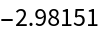The statistic is negative, indicating a downward trend:

 In:=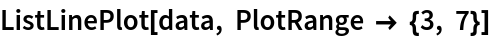Out=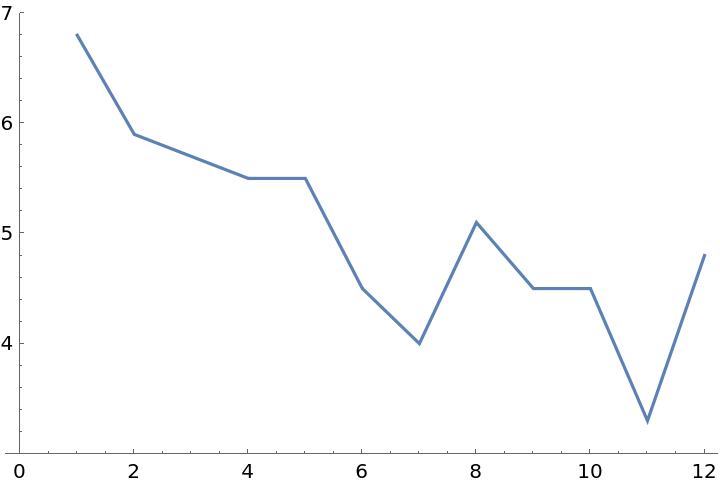### Scope (2)

Various sets of data demonstrating negative, zero and positive trends, along with their corresponding z-statistics:

 In:=Out=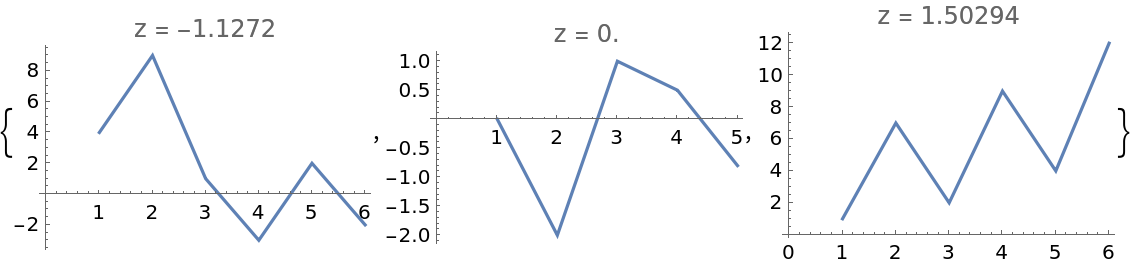Compute the z-statistic of a TimeSeries object:

 In:=Out=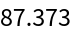### Applications (3)

Use MannKendallZ to determine the general trend of the GDP of Kazakhstan from 1991 to 2019:

 In:=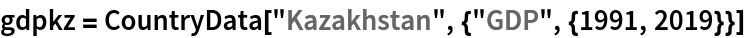Out=In:=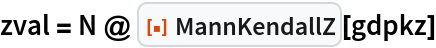Out=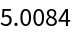The plot confirms the generally positive trend of the data:

 In:=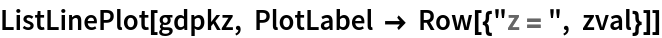Out=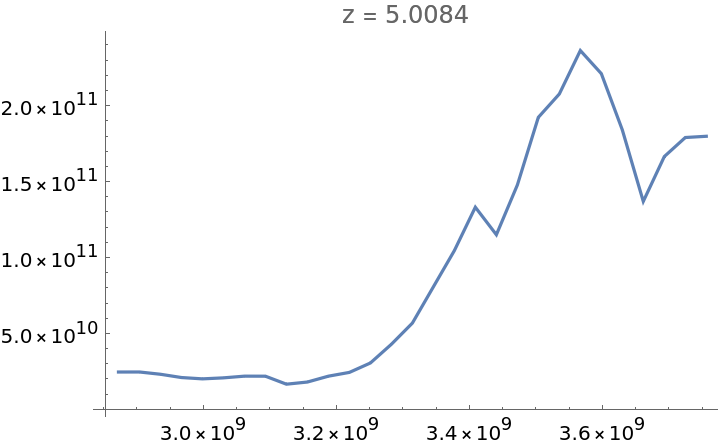Compute the corresponding p-value for a two-sided test:

 In:=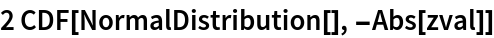Out=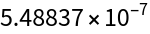## Publisher

Wolfram Summer Camp

## Version History

• 1.0.0 – 13 July 2021